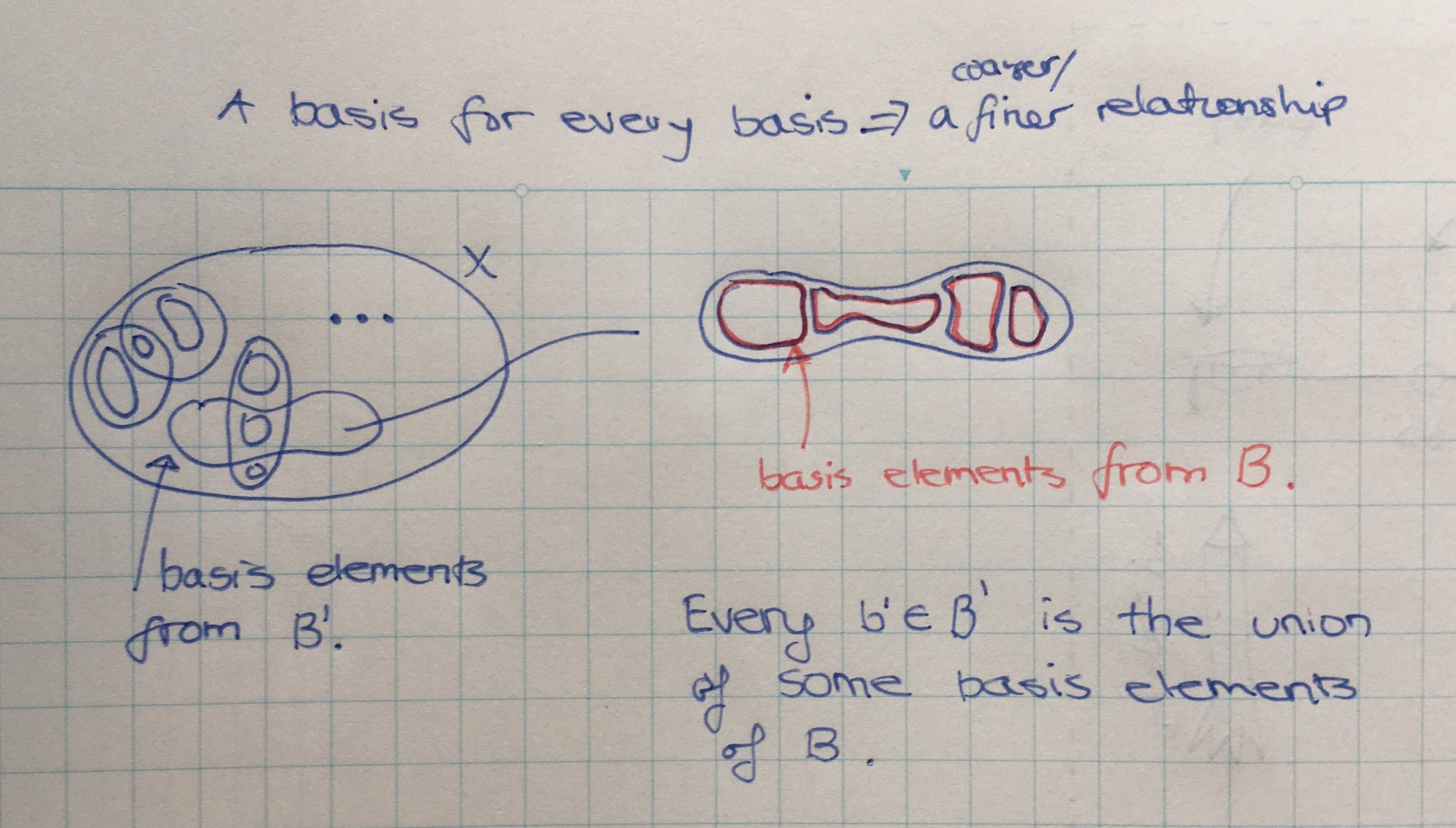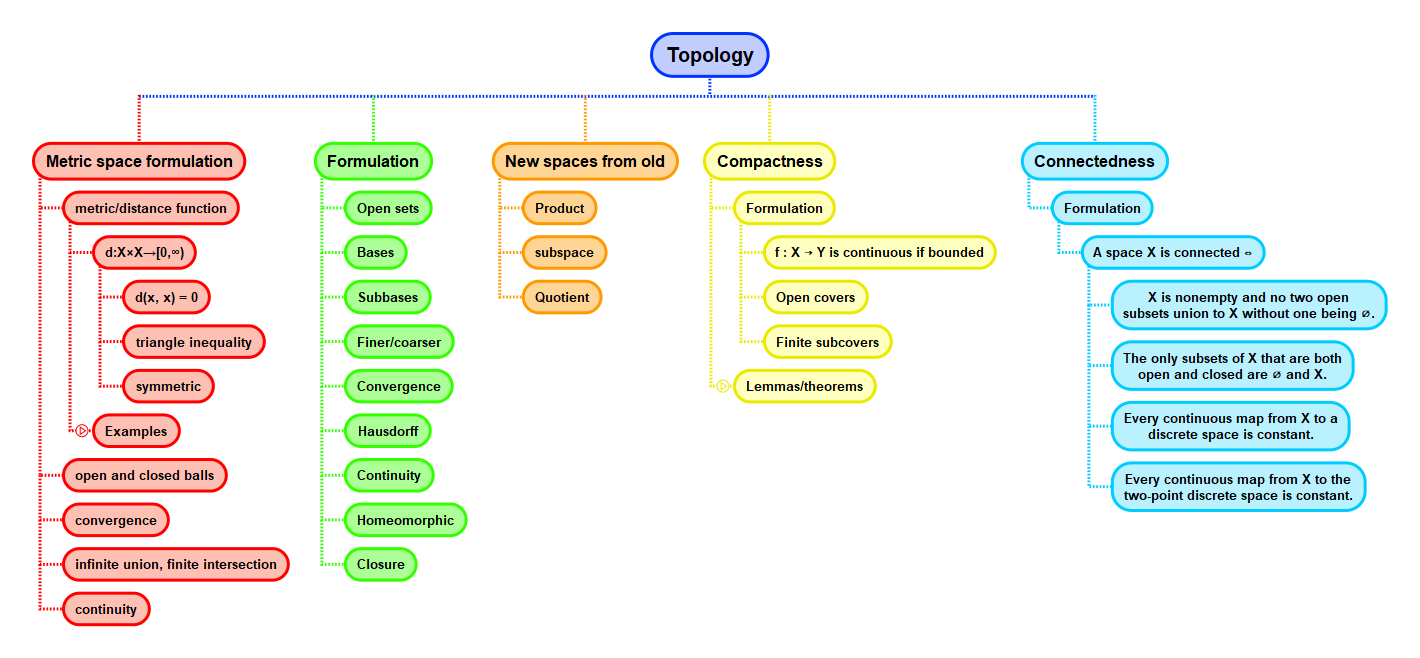Show Question
Math and science::Topology

# Finer and coarser topologies

We say that one topology $$\mathcal{T}$$ on $$X$$ is finer than another topology $$\mathcal{T}'$$ on $$X$$ iff every member of $$\mathcal{T}'$$ is a member of $$\mathcal{T}$$. $$\mathcal{T}'$$ is said to be coarser than $$\mathcal{T}$$.

Stronger and weaker are alternative terminology for finer and coarser.

$$\mathcal{T}$$ is strictly finer iff $$\mathcal{T}'$$ is a proper subset.

Symbolically,

• $$\mathcal{T}$$ is finer than $$\mathcal{T}'$$ ⟺ $$\mathcal{T}' \subseteq \mathcal{T}$$.
• $$\mathcal{T}$$ is strictly finer than $$\mathcal{T}'$$ ⟺ $$\mathcal{T}' \subset \mathcal{T}$$.

#### Lemma. A basis for every basis.

Let $$X$$ be a set. Let $$\mathcal{T}$$ and $$\mathcal{T}'$$ be topologies on $$X$$ with bases $$\mathscr{B}$$ and $$\mathscr{B}'$$ respectively. Then $$\mathcal{T}$$ is finer than $$\mathcal{T}'$$ iff

for each $$x \in X$$ and each basis element $$B' \in \mathscr{B'}$$ containing $$x$$, there is a basis element $$B \in \mathscr{B}$$ such that $$x \in B \subseteq B'$$.

It should be easy to prove this. Refer to Munkres for a proof example.

By this lemma, it can be seen how a topology induced by one metric can be equivalent to a topology induced by another.

#### A basis for every basis: alternative perspective

I think a better way of phasing the second part of the iff relationship is to say: every $$b' \in \mathscr{B'}$$ can be expressed as a union of elements $$b \in \mathscr{B}$$.### Example

For a set $$X$$, the discrete topology is the finest topology while the indiscrete topology is the coarsest topology.

## Context